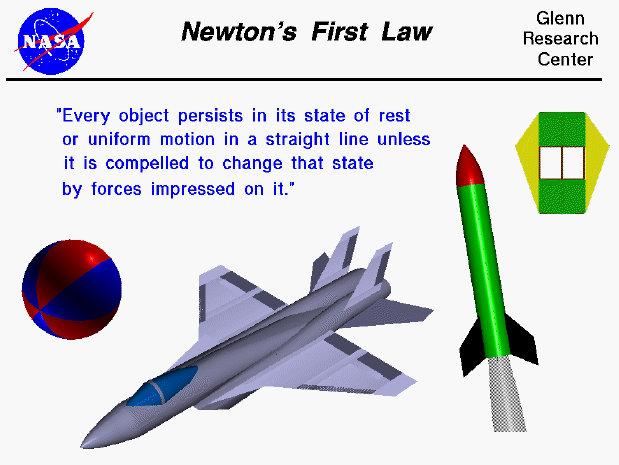Sir Isaac Newton first presented his three laws of motion in the "Principia Mathematica Philosophiae Naturalis" in 1686. His first law states that every object will remain at rest or in uniform motion in a straight line unless compelled to change its state by the action of an external force. This is normally taken as the definition of inertia. The key point here is that if there is no net force resulting from unbalanced forces acting on an object (if all the external forces cancel each other out), then the object will maintain a constant velocity. If that velocity is zero, then the object remains at rest. And if an additional external force is applied, the velocity will change because of the force. The amount of the change in velocity is determined by Newton's second law of motion.

There are many excellent examples of Newton's first law involving aerodynamics. The motion of an airplane when the pilot changes the throttle setting of the engine is described by the first law. The motion of a ball falling down through the atmosphere, or a model rocket being launched up into the atmosphere are both examples of Newton's first law. The motion of a kite when the wind changes can also be described by the first law. We have created separate pages which describe each of these examples in more detail to help you understand this important physical principle.Guided Tours